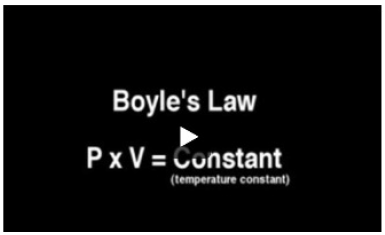# Problem: A certain gas is present in a 15.0 L cylinder at 4.0 atm pressure. If the pressure is increased to 8.0 atm the volume of the gas decreases to 7.5 L. Find the two constants ki, the initial value of k, and kf, the final value of k, to verify whether the gas obeys Boyle’s law by entering the numerical values for ki and kf in the space provided.Express your answers to two significant figures separated by a comma.Watch the video that shows the relationship between pressure and volume for a particular gas. Robert Boyle was the first one to observe the change in the pressure of a gas when its volume is increased or decreased at a constant temperature and the change in the volume of a gas when its pressure is increased or decreased at a constant temperature.

###### FREE Expert Solution
85% (247 ratings)View Complete Written Solution
###### Problem Details

A certain gas is present in a 15.0 L cylinder at 4.0 atm pressure. If the pressure is increased to 8.0 atm the volume of the gas decreases to 7.5 L. Find the two constants ki, the initial value of k, and kf, the final value of k, to verify whether the gas obeys Boyle’s law by entering the numerical values for ki and kf in the space provided.

Watch the video that shows the relationship between pressure and volume for a particular gas. Robert Boyle was the first one to observe the change in the pressure of a gas when its volume is increased or decreased at a constant temperature and the change in the volume of a gas when its pressure is increased or decreased at a constant temperature.